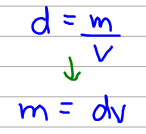# Problem: Mercury poisoning is a debilitating disease that is often fatal. In the human body, mercury reacts with essential enzymes leading to irreversible inactivity of these enzymes. If the amount of mercury in a polluted lake is 0.4 μg Hg/mL, what is the total mass in kilograms of mercury in the lake? (The lake has a surface area of 100 mi2 and an average depth of 20 ft.)

###### FREE Expert Solution
96% (111 ratings)
###### FREE Expert Solution

The density of Hg was given as 0.4 μg Hg/mL. Now the density is basically mass per unit volume so we can calculate mass using the density and the volume.However, we don't have a value for volume so we have to calculate that using the surface area and the depth using the formula below:

96% (111 ratings)###### Problem Details

Mercury poisoning is a debilitating disease that is often fatal. In the human body, mercury reacts with essential enzymes leading to irreversible inactivity of these enzymes. If the amount of mercury in a polluted lake is 0.4 μg Hg/mL, what is the total mass in kilograms of mercury in the lake? (The lake has a surface area of 100 mi2 and an average depth of 20 ft.)

Frequently Asked Questions

What scientific concept do you need to know in order to solve this problem?

Our tutors have indicated that to solve this problem you will need to apply the Density concept. You can view video lessons to learn Density. Or if you need more Density practice, you can also practice Density practice problems.

What professor is this problem relevant for?

Based on our data, we think this problem is relevant for Professor McMahon's class at CANYONS.

What textbook is this problem found in?

Our data indicates that this problem or a close variation was asked in Chemistry: An Atoms First Approach - Zumdahl Atoms 1st 2nd Edition. You can also practice Chemistry: An Atoms First Approach - Zumdahl Atoms 1st 2nd Edition practice problems.